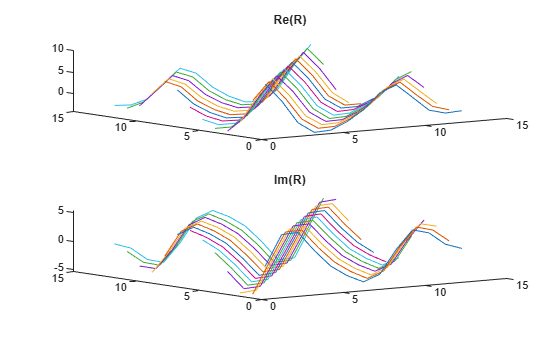corrmtx

Data matrix for autocorrelation matrix estimation

Description

H = corrmtx(x,m) returns an (n+m)-by-(m+1) rectangular Toeplitz matrix H = H such that HH is a biased estimate of the autocorrelation matrix for the input vector x. n is the length of x, m is the prediction model order, and H is the conjugate transpose of H.

example

H = corrmtx(x,m,method) computes the matrix H according to the method specified by method.

[H,r] = corrmtx(___) also returns the (m + 1)-by-(m + 1) autocorrelation matrix estimate r, computed as HH, for any of the previous syntaxes.

Examples

collapse all

Generate a signal composed of three complex exponentials embedded in white Gaussian noise. Compute the data and autocorrelation matrices using the 'modified' method.

n = 0:99;
s = exp(i*pi/2*n)+2*exp(i*pi/4*n)+exp(i*pi/3*n)+randn(1,100);
m = 12;
[X,R] = corrmtx(s,m,'modified');

Plot the real and imaginary parts of the autocorrelation matrix.

[A,B] = ndgrid(1:m+1);
subplot(2,1,1)
plot3(A,B,real(R))
title('Re(R)')
subplot(2,1,2)
plot3(A,B,imag(R))
title('Im(R)')Input Arguments

collapse all

Input data, specified as a vector.

Prediction model order, specified as a positive real integer.

Matrix computation method, specified as 'autocorrelation', 'prewindowed', 'postwindowed', 'covariance' or 'modified'.

• 'autocorrelation': (default) H is the (n + m)-by-(m + 1) rectangular Toeplitz matrix that generates an autocorrelation estimate for the length-n data vector x, derived using prewindowed and postwindowed data, based on an mth-order prediction model. The matrix can be used to perform autoregressive parameter estimation using the Yule-Walker method. For more details, see aryule.

• 'prewindowed': H is the n-by-(m + 1) rectangular Toeplitz matrix that generates an autocorrelation estimate for the length-n data vector x, derived using prewindowed data, based on an mth-order prediction model.

• 'postwindowed': H is the n-by-(m + 1) rectangular Toeplitz matrix that generates an autocorrelation estimate for the length-n data vector x, derived using postwindowed data, based on an mth-order prediction model.

• 'covariance': H is the (nm)-by-(m + 1) rectangular Toeplitz matrix that generates an autocorrelation estimate for the length-n data vector x, derived using nonwindowed data, based on an mth-order prediction model. The matrix can be used to perform autoregressive parameter estimation using the covariance method. For more details, see arcov.

• 'modified': H is the 2(nm)-by-(m + 1) modified rectangular Toeplitz matrix that generates an autocorrelation estimate for the length-n data vector x, derived using forward and backward prediction error estimates, based on an mth-order prediction model. The matrix can be used to perform autoregressive parameter estimation using the modified covariance method. For more details, see armcov.

Output Arguments

collapse all

Data matrix, returned for autocorrelation matrix estimation. The size of H depends on the matrix computation method specified in method.

Biased autocorrelation matrix, returned as a (m + 1)-by-(m + 1) rectangular Toeplitz matrix.

Algorithms

The Toeplitz data matrix computed by corrmtx depends on the method you select. The matrix determined by the autocorrelation (default) method is:

$H=\frac{1}{\sqrt{n}}\left[\begin{array}{ccccc}x\left(1\right)& 0& \cdots & 0& 0\\ x\left(2\right)& x\left(1\right)& \cdots & 0& 0\\ x\left(3\right)& x\left(2\right)& \cdots & 0& 0\\ ⋮& ⋮& \ddots & ⋮& ⋮\\ x\left(m\right)& x\left(m-1\right)& \cdots & x\left(1\right)& 0\\ x\left(m+1\right)& x\left(m\right)& \cdots & x\left(2\right)& x\left(1\right)\\ x\left(m+2\right)& x\left(m+1\right)& \cdots & x\left(3\right)& x\left(2\right)\\ ⋮& ⋮& \ddots & ⋮& ⋮\\ x\left(n-1\right)& x\left(n-2\right)& \cdots & x\left(n-m\right)& x\left(n-m-1\right)\\ x\left(n\right)& x\left(n-1\right)& \cdots & x\left(n-m+1\right)& x\left(n-m\right)\\ 0& x\left(n\right)& \cdots & x\left(n-m+2\right)& x\left(n-m+1\right)\\ ⋮& ⋮& ⋰& ⋮& ⋮\\ 0& 0& \cdots & x\left(n-1\right)& x\left(n-2\right)\\ 0& 0& \cdots & x\left(n\right)& x\left(n-1\right)\\ 0& 0& \cdots & 0& x\left(n\right)\end{array}\right].$

In the matrix, m is the same as the input argument m to corrmtx and n is length(x). Variations of this matrix are used to return the output H of corrmtx for each method:

• 'autocorrelation' — (default) H = H.

• 'prewindowed'H is the n-by-(m + 1) submatrix of H whose first row is [x(1) … 0] and whose last row is [x(n) … x(nm)].

• 'postwindowed'H is the n-by-(m + 1) submatrix of H whose first row is [x(m + 1) … x(1)] and whose last row is [0 … x(n)].

• 'covariance'H is the (nm)-by-(m + 1) submatrix of H whose first row is [x(m + 1) … x(1)] and whose last row is [x(n) … x(nm)].

• 'modified'H is the 2(nm)-by-(m + 1) matrix Hmod defined by

${H}_{\mathrm{mod}}=\frac{1}{\sqrt{2\left(n-m\right)}}\left[\begin{array}{ccc}x\left(m+1\right)& \cdots & x\left(1\right)\\ ⋮& ⋰& ⋮\\ x\left(n\right)& \cdots & x\left(n-m\right)\\ {x}^{\ast }\left(1\right)& \cdots & {x}^{\ast }\left(m+1\right)\\ ⋮& \ddots & ⋮\\ {x}^{\ast }\left(n-m\right)& \cdots & {x}^{\ast }\left(n\right)\end{array}\right].$

 Marple, S. Lawrence. Digital Spectral Analysis: With Applications. Prentice-Hall Signal Processing Series. Englewood Cliffs, N.J: Prentice-Hall, 1987.